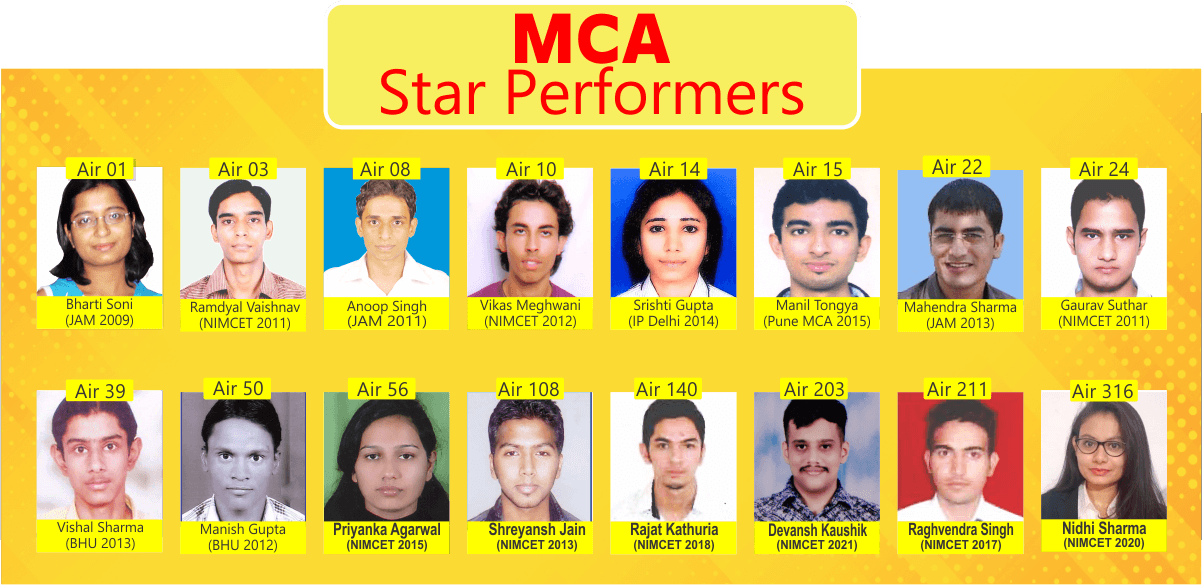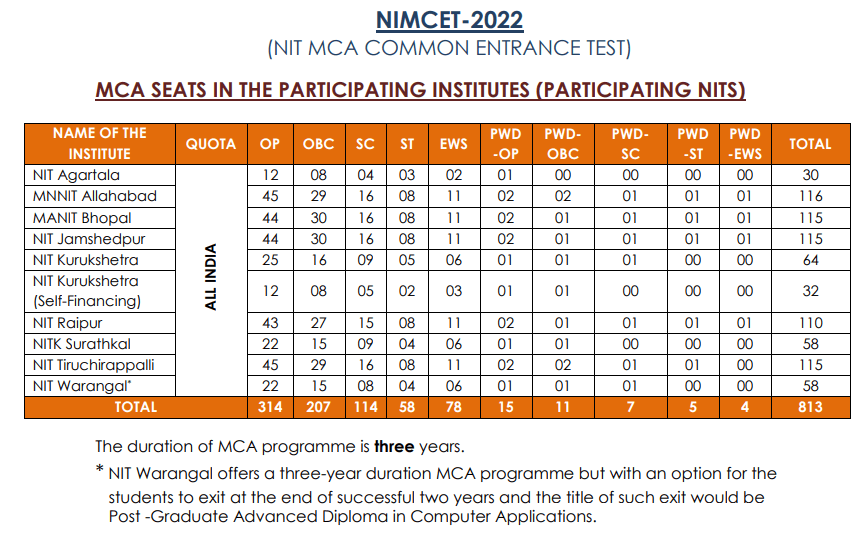## Join NIMCET 23 course

#### NIMCET:

NIMCET is a national level entrance test conducted by NITs for admission to their MCA program. The admission to MCA program offered by all the NITs that participate in NIMCET is based on the rank obtained in NIMCET.

#### Duration of MCA Course:

The duration of MCA programme at NIT Patna is two years.
NIT Warangal offers a three-year duration MCA programme but with an option for the students to exit at the end of successful two years and the title of such exit would be Post Graduate Advanced Diploma in Computer Applications. The duration of MCA programme is three years in the remaining Participating Institutes.
(Please refer to the websites of the respective Institutes for further information)

#### Exam Pattern:

NIMCET test will be conducted with only one question paper containing 120 multiple choice questions covering the following subjects:

Mathematics: 50 questions
Analytical Ability & Logical Reasoning: 40 questions
Computer Awareness:  10 questions
General English: 20 questions

In the final score the weightage of Higher Mathematics, Reasoning, Computers and English questions are 12, 6, 8, 4. That makes the total marks in the paper as 50×12 + 40×6 + 10×8 + 20×4 = 1000. Students must give adequate time for practicing higher mathematics as it constitutes 60% of the total marks.

Ranking will be based on total weighted marks obtained by a candidate.

In case of a tie, it will be resolved based on weighted marks in Mathematics, then on weighted marks in Analytical Ability & Logical reasoning and then on weighted marks in Computer Awareness.

In case the tie is not resolved by the criteria stated in the above paragraph, then it will be resolved by age i.e., in favour of older candidate.#### DETAILED SYLLABUS :

MATHEMATICS :

Set Theory: Concepts of sets – Union – Intersection – Cardinality – Elementary counting permutations and combinations

Probability and Statistics: Basic concepts of probability theory, Averages, Dependent and independent events, frequency distributions, measures of central tendencies and dispersions.

Algebra: Fundamental operations in Algebra, Expansions, Factorization, simultaneous linear/quadratic equations, indices, logarithms, arithmetic, geometric and harmonic progressions, determinants and matrices.

Coordinate Geometry: Rectangular Cartesian coordinates, distance formulae, equation of a line, intersection of lines, pair of straight lines, equations of a circle, parabola, ellipse, and hyperbola.

Calculus: Limit of functions, Continuous function, Differentiation of function, tangents and normals, simple examples of maxima and minima. Integration of function by parts, by substitution and by partial fraction; Definite integrals, Applications of Definite Integrals to areas.

Vectors: Position vector, addition and subtraction of vectors, scalar and vector products and their applications to simple geometrical problems and mechanics.

Trigonometry: Simple identities, trigonometric equations, properties of triangles, solution of triangles, heights and distances, General solutions of trigonometric equations.

ANALYTICAL ABILITY AND LOGICAL REASONING :

The questions in this section will cover logical reasoning and quantitative aptitude. Some of the questions will be on comprehension of a logical situation and questions based on the facts given in the passage.

COMPUTER AWARENESS :

Computer Basics: Organization of a Computer, Central Processing Unit (CPU), Structure of instructions in CPU, input/output devices, computer memory, back-up devices. Representation of characters, integers and fractions, binary and hexadecimal representations, Binary Arithmetic: Addition, subtraction, multiplication, division, simple arithmetic and two’s complement arithmetic, floating point representation of numbers, Boolean algebra, truth tables, Venn diagrams.

GENERAL ENGLISH:

Questions in this section will be designed to test the candidates’ general understanding of the English language. There will be questions on the following topics.

Comprehension, Vocabulary, Basic English Grammar (like usage of correct forms of verbs, prepositions and articles), Word power, Synonyms and Antonyms, Meanings of words and phrases, Technical writing.

#### Number of seats:

Here is the number of seats in participating NITS.#### Participating INSTITUTES:

NIT Agartala, MNNIT Allahabad, MANIT Bhopal, MNIT Suratkal, NIT Jamshedpur, NIT Kurukshetra, NIT Raipur, NIT Trichy, NIT Warangal

For further details:

https://nimcet.in/